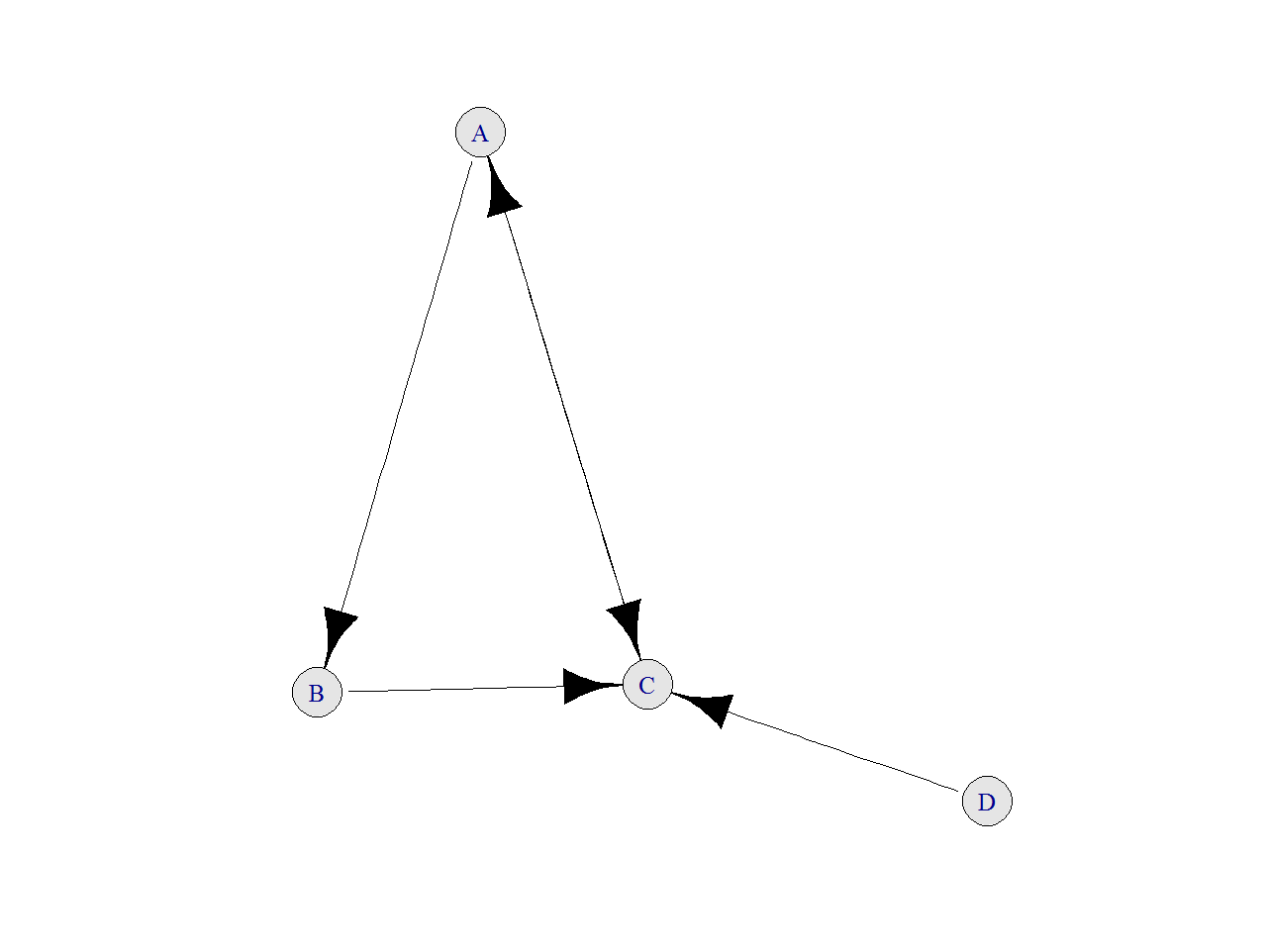# PageRank For SQL Lovers

If you’re changing the world, you’re working on important things. You’re excited to get up in the morning (Larry Page, CEO and Co-Founder of Google)

This is my particular tribute to one of the most important, influential and life-changer R packages I have discovered in the last times: `sqldf `package.

Because of my job, transforming data through SQL queries is very natural for me. This, together with the power of R made this package indispensable for me since I knew of its existence.

Imagine you have a directed graph like this:Given a vertex V, these are the steps to calculate its PageRank, lets call it PR(V):

• Initialize PR(V) to some value (I do it to 1 in my script)
• Iterate this formula until converges: `PR(V)=(1-d)+d*(PR(T1)/C(T1)+ ... +PR(Tn)/C(Tn))` where `Ti` are the vertex that point to V and `C(Ti)` is the number of edges going out of `Ti`

After doing this, result is:Following you can find my code to do it with `sqldf`, which is quite simple from my point of view. I am pretty sure there must be some package which calculates PageRank but the main goal of this post is to show how easy is to calculate it with two simple queries, no more. The example is taken from here, where you can find a good explanation of how PageRank works:

```require(sqldf)
require(igraph)
net=data.frame(origin=c("A","A","B","C","D"), end=c("C","B","C","A","C"))
par(family="serif", cex=1, ps=25, bg="white", col.lab="black", col.axis="black")
plot(graph.edgelist(as.matrix(net)), edge.arrow.size=1, vertex.color="gray90", edge.color="black")
#Initialization
netou=sqldf("SELECT origin, COUNT(*) outs FROM net GROUP BY 1")
netpr=sqldf("SELECT origin vertex, 1.0 pagerank FROM net UNION SELECT end, 1.0 FROM net")
for (i in 1:50)
{
netx1=sqldf("SELECT vertex, pagerank/outs factor FROM netou a INNER JOIN netpr b ON (a.origin = b.vertex)")
netpr=sqldf("SELECT a.vertex, 0.15+SUM(0.85*COALESCE(factor,0)) AS pagerank
FROM netpr a LEFT OUTER JOIN net b ON (a.vertex = b.end) LEFT OUTER JOIN netx1 c
ON (b.origin = c.vertex) GROUP BY 1")
}
g=graph.edgelist(as.matrix(net))
names=data.frame(vertex=V(g)\$name)
V(g)\$name=sqldf("SELECT a.vertex||' (PR='||ROUND(b.pagerank,2)||')' as name from names a inner join netpr b ON (a.vertex=b.vertex)")\$name
plot(g, edge.arrow.size=1, vertex.color="gray90", edge.color="black")
```

## 2 thoughts on “PageRank For SQL Lovers”

1.Christian says:

Also, I’m interested in ranking soccer teams using a page rank from 0 to 1. Do you think this is easy to do?

1.aschinchon says:

It should be once you define links between teams. Did you?